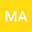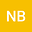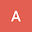Lie group method for analysing the generalized heat transfer mathematical model for Lake Tahoe
•••• +1
• m.b. abd-el-malek,
• Amr AMIN,
• Anood HANAFY
m.b. abd-el-malek
Alexandria University

Corresponding Author:minab@aucegypt.edu

Author ProfileAlexandria University
Author ProfileAmr AMIN
Alexandria University
Author ProfileAnood HANAFY
Alexandria University
Author Profile## Abstract

The one dimensional time-dependant heat transfer equation in a vertical direction is introduced in terms of general formula of density and thermal conductivity. One-parameter Lie symmetry group transformation is used to determine the suitable forms of density and thermal conductivity of the water based on experimental measurements. The general equation is investigated again using Lie group analysis after substitution by the two possible cases of water density and thermal conductivity from the first part. The obtained partial differential equation is solved numerically using explicit 4th and 5th Runge-Kutta formula or analytically if it is possible by assuming the physical parameters of Lake Tahoe in the Sierra Nevada of the United States. The temperature distribution across the lake depth from each case is illustrated graphically to indicate the thermal stratification phenomenon of lakes.